Showing:

0

460

3mos ago

1

0

# NumPyCNN: Implementing Convolutional Neural Networks From Scratch

NumPyCNN is a Python implementation for convolutional neural networks (CNNs) from scratch using NumPy.

IMPORTANT If you are coming for the code of the tutorial titled Building Convolutional Neural Network using NumPy from Scratch, then it has been moved to the TutorialProject directory on 20 May 2020.

The project has a single module named `cnn.py` which implements all classes and functions needed to build the CNN.

It is very important to note that the project only implements the forward pass of training CNNs and there is no learning algorithm used. Just the learning rate is used to make some changes to the weights after each epoch which is better than leaving the weights unchanged.

The project can be used for classification problems where only 1 class per sample is allowed.

The project will be extended to train CNN using the genetic algorithm with the help of a library named PyGAD. Check the library's documentation at Read The Docs: https://pygad.readthedocs.io

# Donation

You can donate via Open Collective: opencollective.com/pygad.

# Installation

For Windows, issue the following command:

``````pip3 install pygad
``````

PyGAD is developed in Python 3.7.3 and depends on NumPy for creating and manipulating arrays and Matplotlib for creating figures. The exact NumPy version used in developing PyGAD is 1.16.4. For Matplotlib, the version is 3.1.0.

The source code of the PyGAD' modules is found in the following GitHub projects:

The documentation of the PyGAD library is available at Read The Docs at this link: https://pygad.readthedocs.io. It discusses the modules supported by PyGAD, all its classes, methods, attribute, and functions. For each module, a number of examples are given.

If there is an issue using PyGAD, feel free to post at issue in this GitHub repository https://github.com/ahmedfgad/GeneticAlgorithmPython or by sending an e-mail to ahmed.f.gad@gmail.com.

If you built a project that uses PyGAD, then please drop an e-mail to ahmed.f.gad@gmail.com with the following information so that your project is included in the documentation.

• Project title
• Brief description

The next figure lists the different stages in the lifecycle of an instance of the `pygad.GA` class. Note that PyGAD stops when either all generations are completed or when the function passed to the `on_generation` parameter returns the string `stop`.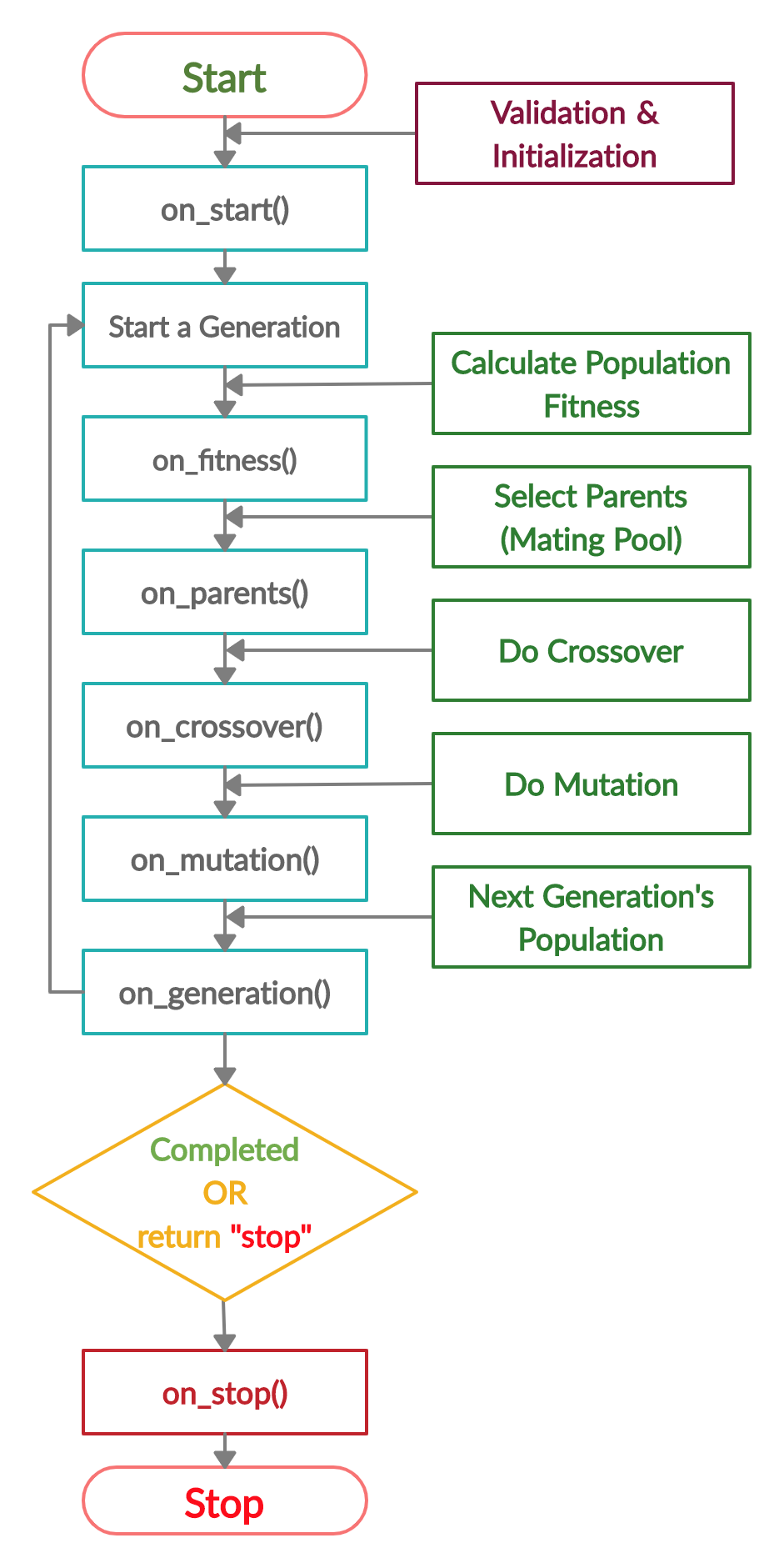The next code implements all the callback functions to trace the execution of the genetic algorithm. Each callback function prints its name.

``````import pygad
import numpy

function_inputs = [4,-2,3.5,5,-11,-4.7]
desired_output = 44

def fitness_func(solution, solution_idx):
output = numpy.sum(solution*function_inputs)
fitness = 1.0 / (numpy.abs(output - desired_output) + 0.000001)
return fitness

fitness_function = fitness_func

def on_start(ga_instance):
print("on_start()")

def on_fitness(ga_instance, population_fitness):
print("on_fitness()")

def on_parents(ga_instance, selected_parents):
print("on_parents()")

def on_crossover(ga_instance, offspring_crossover):
print("on_crossover()")

def on_mutation(ga_instance, offspring_mutation):
print("on_mutation()")

def on_generation(ga_instance):
print("on_generation()")

def on_stop(ga_instance, last_population_fitness):
print("on_stop()")

num_parents_mating=5,
fitness_func=fitness_function,
sol_per_pop=10,
num_genes=len(function_inputs),
on_start=on_start,
on_fitness=on_fitness,
on_parents=on_parents,
on_crossover=on_crossover,
on_mutation=on_mutation,
on_generation=on_generation,
on_stop=on_stop)

ga_instance.run()
``````

Based on the used 3 generations as assigned to the `num_generations` argument, here is the output.

``````on_start()

on_fitness()
on_parents()
on_crossover()
on_mutation()
on_generation()

on_fitness()
on_parents()
on_crossover()
on_mutation()
on_generation()

on_fitness()
on_parents()
on_crossover()
on_mutation()
on_generation()

on_stop()
``````

# Example

Check the PyGAD's documentation for information about the implementation of this example.

``````import numpy

sample_shape = train_inputs.shape[1:]
num_classes = 4

kernel_size=3,
previous_layer=input_layer,
activation_function=None)
previous_layer=relu_layer1,
stride=2)

kernel_size=3,
previous_layer=average_pooling_layer,
activation_function=None)
previous_layer=relu_layer2,
stride=2)

kernel_size=3,
previous_layer=max_pooling_layer,
activation_function=None)
previous_layer=relu_layer3,
stride=2)

previous_layer=flatten_layer,
activation_function="relu")
previous_layer=dense_layer1,
activation_function="softmax")

epochs=1,
learning_rate=0.01)

model.summary()

model.train(train_inputs=train_inputs,
train_outputs=train_outputs)

predictions = model.predict(data_inputs=train_inputs)
print(predictions)

num_wrong = numpy.where(predictions != train_outputs)
num_correct = train_outputs.size - num_wrong.size
accuracy = 100 * (num_correct/train_outputs.size)
print("Number of correct classifications : {num_correct}.".format(num_correct=num_correct))
print("Number of wrong classifications : {num_wrong}.".format(num_wrong=num_wrong.size))
print("Classification accuracy : {accuracy}.".format(accuracy=accuracy))
``````

There are different resources that can be used to get started with the building CNN and its Python implementation.

## Tutorial: Implementing Genetic Algorithm in Python

To start with coding the genetic algorithm, you can check the tutorial titled Genetic Algorithm Implementation in Python available at these links:

This tutorial is prepared based on a previous version of the project but it still a good resource to start with coding the genetic algorithm.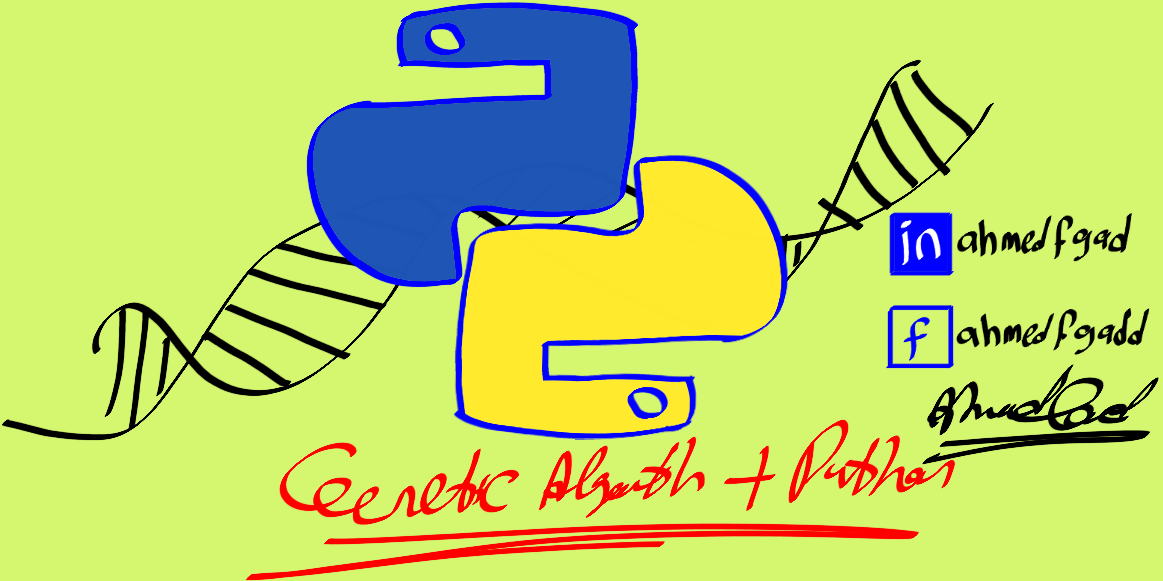## Tutorial: Introduction to Genetic Algorithm

Get started with the genetic algorithm by reading the tutorial titled Introduction to Optimization with Genetic Algorithm which is available at these links: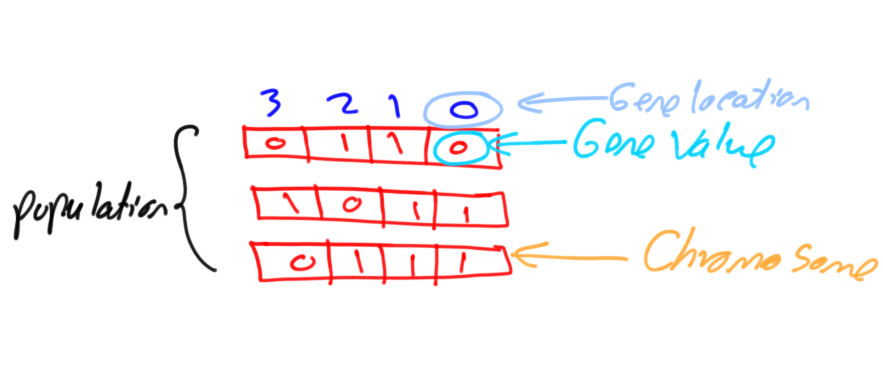## Tutorial: Build Neural Networks in Python

Read about building neural networks in Python through the tutorial titled Artificial Neural Network Implementation using NumPy and Classification of the Fruits360 Image Dataset available at these links: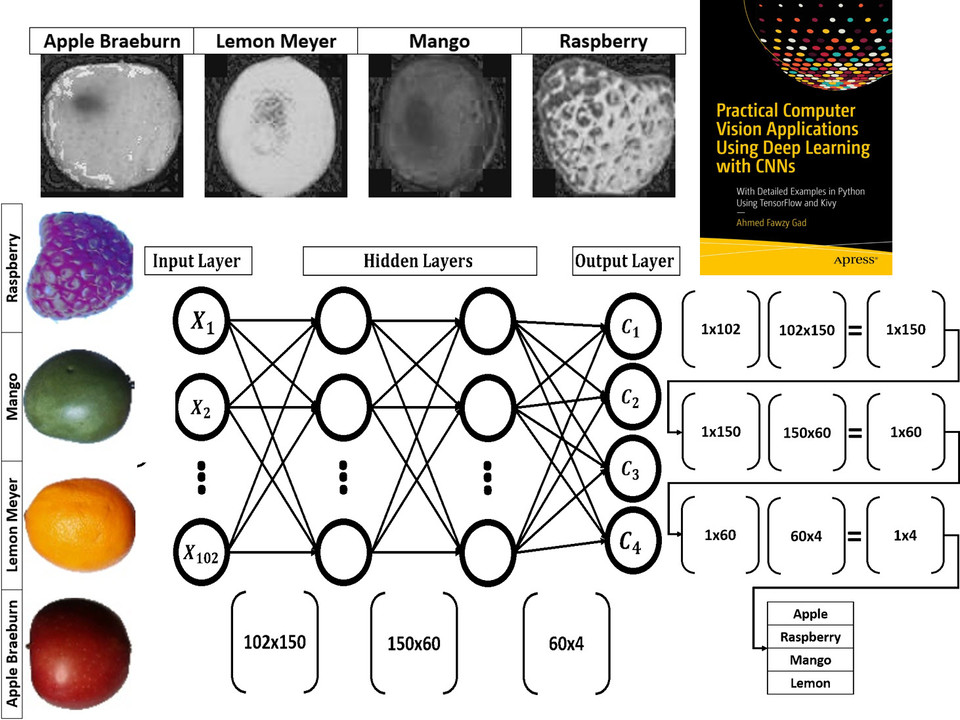## Tutorial: Optimize Neural Networks with Genetic Algorithm

Read about training neural networks using the genetic algorithm through the tutorial titled Artificial Neural Networks Optimization using Genetic Algorithm with Python available at these links: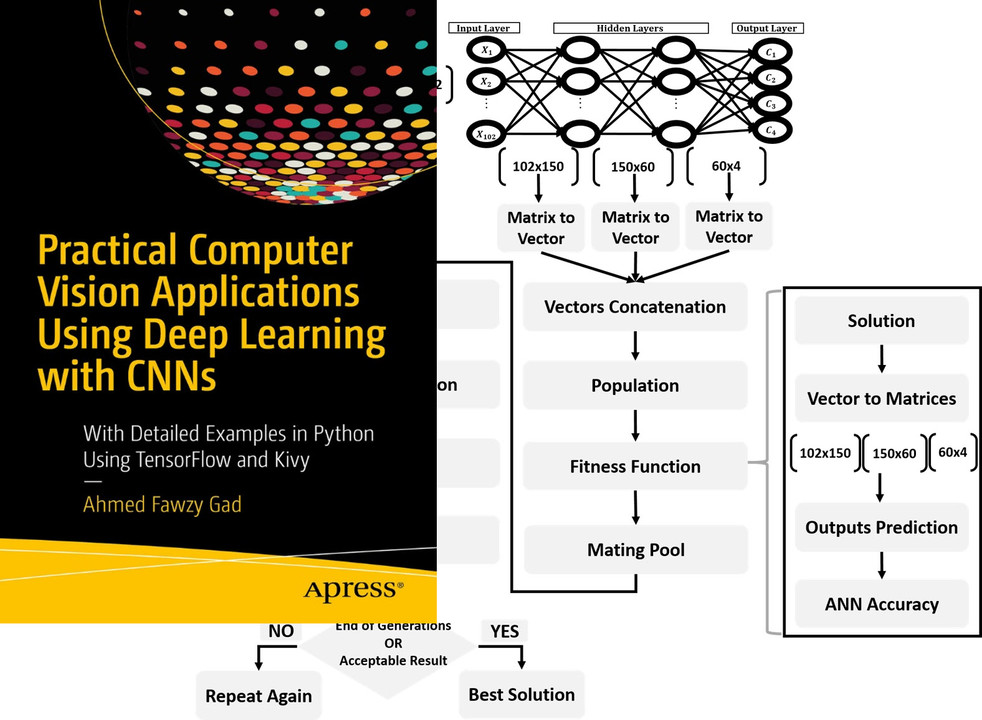## Tutorial: Building CNN in Python

To start with coding the genetic algorithm, you can check the tutorial titled Building Convolutional Neural Network using NumPy from Scratch available at these links:

This tutorial) is prepared based on a previous version of the project but it still a good resource to start with coding CNNs.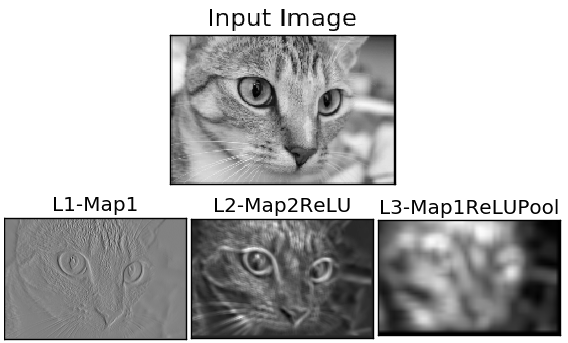## Tutorial: Derivation of CNN from FCNN

Get started with the genetic algorithm by reading the tutorial titled Derivation of Convolutional Neural Network from Fully Connected Network Step-By-Step which is available at these links: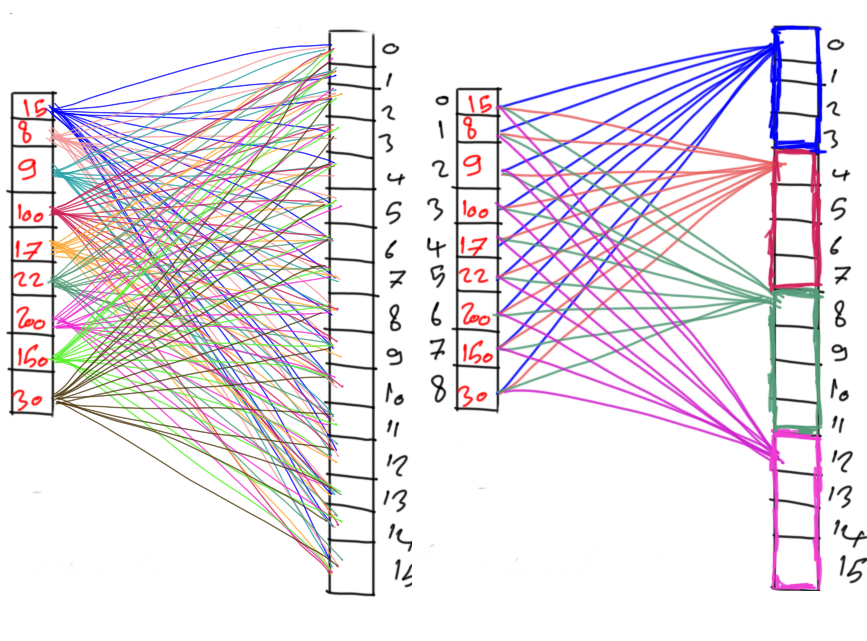## Book: Practical Computer Vision Applications Using Deep Learning with CNNs

You can also check my book cited as Ahmed Fawzy Gad 'Practical Computer Vision Applications Using Deep Learning with CNNs'. Dec. 2018, Apress, 978-1-4842-4167-7 which discusses neural networks, convolutional neural networks, deep learning, genetic algorithm, and more.

Find the book at these links: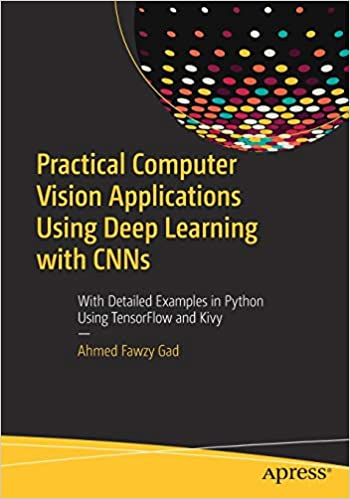# Citing PyGAD - Bibtex Formatted Citation

``````@misc{gad2021pygad,
title={PyGAD: An Intuitive Genetic Algorithm Python Library},
year={2021},
eprint={2106.06158},
archivePrefix={arXiv},
primaryClass={cs.NE}
}
``````

## Rate & Review

Great Documentation0
Easy to Use0
Performant0
Highly Customizable0
Bleeding Edge0
Responsive Maintainers0
Poor Documentation0
Hard to Use0
Slow0
Buggy0
Abandoned0
Unwelcoming Community0
100